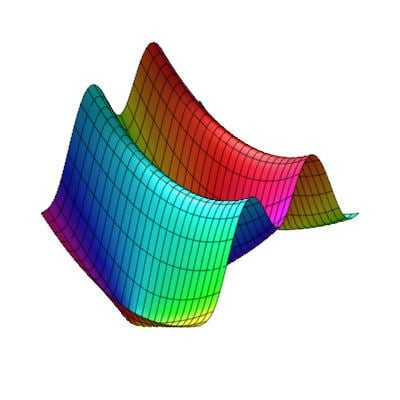# Lommel function

Updated onThe Lommel differential equation is an inhomogeneous form of the Bessel differential equation:

z 2 d 2 y d z 2 + z d y d z + ( z 2 ν 2 ) y = z μ + 1 .

Two solutions are given by the Lommel functions sμ,ν(z) and Sμ,ν(z), introduced by Eugen von Lommel (1880),

s μ , ν ( z ) = π 2 [ Y ν ( z ) 0 z x μ J ν ( x ) d x J ν ( z ) 0 z x μ Y ν ( x ) d x ] S μ , ν ( z ) = s μ , ν ( z ) + 2 μ 1 Γ ( μ + ν + 1 2 ) Γ ( μ ν + 1 2 ) ( sin [ ( μ ν ) π 2 ] J ν ( z ) cos [ ( μ ν ) π 2 ] Y ν ( z ) )

where Jν(z) is a Bessel function of the first kind, and Yν(z) a Bessel function of the second kind.

## References

Similar Topics
Falling Overnight
Sam McMahon